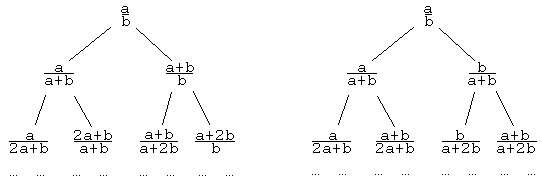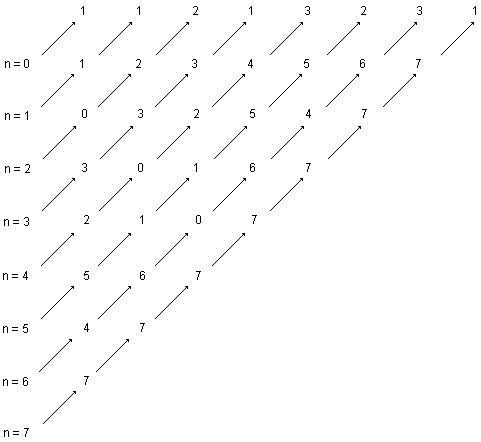# Fractions on a Binary Tree II

We have already discussed an instance of a binary tree related to the Stern-Brocot tree. A fraction $\frac{a}{b}$ on the tree served a parent to two other fractions: $\displaystyle\frac{a}{a + b}$ and $\displaystyle\frac{b}{a + b}.$ At the time we only considered the case where $a \lt b.$ With the root equal to $\displaystyle\frac{1}{2},$ the tree has been populated by all positive fractions less than $1.$ Each fraction on the tree appeared in lowest terms. Had we started with the root of $\displaystyle\frac{2}{1},$ we would have obtained a tree (of reciprocals) populated by all fractions greater than $1,$ again all in lowest terms.

By a little change of procedure Calkin and Wilf arrived at a tree with many additional and no less remarkable features. In the modified tree, each fraction $\displaystyle\frac{a}{b}$ has two children, which in order of their appearance on the tree are $\displaystyle\frac{a}{a + b},$ as before, and $\displaystyle\frac{a + b}{b},$ which is the reciprocal of the second term in the previous construct. The diagram below depicts the two trees -- the new and the old -- rooted at the same fraction $\displaystyle\frac{a}{b}:$

 (1)In terms of functions $f_{L}$ and $f_{R},$ defined for the fractions on the Stern-Brocot tree, the new algorithm generates two children for any fraction $\displaystyle\frac{a}{b}:$ $f_{L}(\frac{a}{b})$ and $\displaystyle\frac{1}{f_{R}(\frac{a}{b})}.$ These are exactly the fractions generated on the Stern-Brocot tree, albeit, in an entirely different order. Thus we may claim that row by row the two trees contain exactly same fractions.

Starting with $\displaystyle\frac{a}{b} = \frac{1}{1},$ the new tree appears as

 (2)As the Stern-Brocot tree, it, too, contains all positive rational numbers and each of them appears exactly once. Calkin and Wilf noticed that in each row any two neighboring fraction have an additional property:

(3)

The denominator of the left neighbor coincides with the numerator of the right neighbor.

Also, $1$ being the numerator of the first fraction in each row as well as the denominator of the last one, (3) holds for the sequence of fractions obtained by writing the tree terms row by row:

(4)

$\displaystyle\frac{1}{1},\,\frac{1}{2},\,\frac{2}{1},\,\frac{1}{3},\,\frac{3}{2},\,\frac{2}{3},\,\frac{3}{1},\,\frac{1}{4},\,\frac{4}{3},\,\frac{3}{5},\,\frac{5}{2},\,\frac{2}{5},\,\frac{5}{3},\,\frac{3}{4},\,\frac{4}{1},\,\ldots$

To prove (3), first note that the property is obvious for two immediate descendants of the same parent. Two neighbors that are not siblings but share a grandparent also have the same property, see (1). In general, two neighboring fractions that are not siblings, descend from two neighboring fractions in the row above. The situation calls for an inductive argument. Assume, for the sake of induction, that the two parents are $\displaystyle\frac{a}{b}$ and $\displaystyle\frac{b}{c}.$ Their four children then are

$\displaystyle\frac{a}{a + b},\,\frac{a + b}{b},\,\frac{b}{b + c},\,\frac{b + c}{c}$,

and the inductive step follows. A look at the first rows of the tree (2) supplies a base for induction. The proof is complete. (An inductive argument based on $\displaystyle f_{R}(\frac{b}{a}) = \frac{1}{f_{L}(\frac{a}{b})}$ shows that each row of the tree has central symmetry.)

Based on (3), the fractions in (4) can be written as $\displaystyle\frac{q(n)}{q(n + 1)},$ $n \ge 0,$ where $q(n)$ is the sequence of numerators

(5)

$1$, $1$, $2$, $1$, $3$, $2$, $3$, $1$, $4$, $3$, $5$, $2$, $5$, $3$, $4,$ $\ldots,$

with $q(0) = q(1) = 1,$ $q(2) = 2,$ $q(3) = 1,$ etc. (The sequence (5) is listed as #A002487 in the On-Line Encyclopedia of Integer Sequences, where it's called Stern's diatomic series. The sequence is remarkable in its own right.)

Function $q(n)$ provides an effective way for enumeration of rational numbers. For $n = 0, 1, 2, \ldots,$ fraction $\#n$ equals $\displaystyle\frac{q(n)}{q(n+1)}.$ That simple.

The sequence (5) has been discovered several times over. E. W. Dijkstra described it in a letter to a friend in 1976. Then later same year he learned that function q(n) has been investigated by G. de Rham yet in 1947. Calkin and Wilf proved that $q(n)$ gives a number of hyperbinary representations of the number $n.$ (In other words, $q(n)$ is the number of ways of writing the integer n as a sum of powers of $2,$ each power being used at most twice (i.e., once more than the legal limit for binary expansions).) Berlekamp et al (1981) identified $q(n)$ with the number of distinct nim-sums of two numbers that add up in the ordinary sense to the number n. Hence, the sequence (5) can be read from an XOR table: $q(n)$ is the number of distinct terms on the $n^{th}$ SW-NE diagonal of the XOR table:The proof of the latter two assertions is based on the fact that function $q(n)$ satisfies a recurrence relation:

(6)

\begin{align} q(2n + 1) &= q(n),\\ q(2n + 2) &= q(n) + q(n + 1),\,n = 0, 1, \ldots \end{align}

(This is because the children of $\displaystyle\frac{q(n)}{q(n + 1)}$ are $\displaystyle\frac{q(2n + 1)}{q(2n + 2)}$ and $\displaystyle\frac{q(2n + 2)}{q(2n + 3)}.$ As it happens, we have the following

### Proposition

1. The number $b(n)$ of hyperbinary representations of $n$ satisfies the recurrence (6) with the same initial condition, $b(0) = 1.$

2. The number $x(n)$ of distinct nim-sums $k \oplus m$, where $k + m = n,$ satisfies the recurrence (6) with the same initial condition, $x(0) = 1.$

### Proof

1. Now, the binary and hence any hyperbinary expansion of an odd number $2n + 1$ ends in $1.$ It follows by first subtracting $1$ and then dividing by $2$ that there is a 1-1 correspondence between the hyperbinary expansions of $2n + 1$ and $n.$ Thus $b(2n + 1) = b(n).$ Furthermore, $b(2n + 2) = b(n) + b(n + 1),$ because a hyperbinary expansion of $2n + 2$ might have either two $1\mbox{'s}$ or no $1\mbox{'s}$ in it. If it has two $1\mbox{'s}$, then by deleting them and dividing by $2$ we'll get an expansion of $n.$ If it has no $1\mbox{'s}$, then by just dividing by $2$ we get an expansion of $n+1.$

2. If $k + m = 2n + 1,$ then the binary expansion of one of $k$ or $m$ ends in $1,$ the other in $0.$ By removing this one and dividing by $2,$ we'll get $k_{1} + m_{1} = n$, for some $k_{1}$ and $m_{1}$ such that $k_{1} \oplus m_{1} = k \oplus m.$ If $k + m = 2n + 2,$ then k and m have binary expansions that either both end in 1 or both end in 0. In the first case, asas before, we find $k_{1} + m_{1} = n$ and also $k_{1} \oplus m_{1} = k \oplus m.$ In the second case, $k_{1} + m_{1} = n + 1$ and still $k_{1} \oplus m_{1} = k \oplus m.$"Playing with figures" on Ascension day 1976 E. W. Dijkstra came across a shifted function (which he called $fusc$):

(6')

\begin{align} fusc(1) &= 1,\\ fusc(2n) &= fusc(n),\\ fusc(2n + 1) &= fusc(n) + fusc(n + 1),\,n = 1, \ldots \end{align}

so that $fusc(k) = q(k - 1),$ $k = 1, 2, 3, \ldots$ Compatible with (6'), Dijkstra also defines $fusc(0) = 0.$ He derived an iterative algorithm to compute $fusc(n)$ and, based on it, has established several properties of the function. The algorithm is cute:

 k = n a = 1 b = 0 while (k > 0 ) {   if (k is even) {     k = k/2     a = a + b   } else {     k = (k-1)/2     b = a + b   } } fusc(n) = b 

Function fusc has the following properties:

1. For $n$ odd, let $m$ be obtained from n by inverting the internal (all, but the first and the last) digits of the binary representation of $n$ $(0$ to $1,$ $1$ to $0).$ Then $fusc(m) = fusc(n).$

2. Let the binary representation of $m$ be the reverse (written in the reverse order) of the binary representation of $n.$ Then $fusc(m) = fusc(n).$

3. $fusc(n)$ is even iff $3|n.$ (Proof)## References

1. E. R. Berlekamp, J. H. Conway, R. K. Guy, Winning Ways for Your Mathematical Plays, Volume 1, A K Peters, 2001, pp. 115-116.
2. E. W. Dijkstra, An exercise for Dr. R. M. Burstall, published in Edsger W. Dijkstra, Selected Writings on Computing: A Personal Perspective, Springer-Verlag, 1982, pp. 215-216.
3. E. W. Dijkstra, More about the function "fusc", published in Edsger W. Dijkstra, Selected Writings on Computing: A Personal Perspective, Springer-Verlag, 1982, pp. 230-232.
4. N. Calkin, H. S. Wilf, Recounting the Rationals, Am Math Monthly, Vol. 107 (2000), pp. 360-363.Stern-Brocot Tree### Countability of Rational Numbers